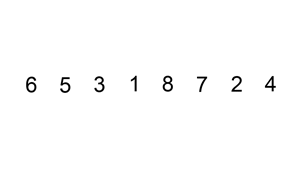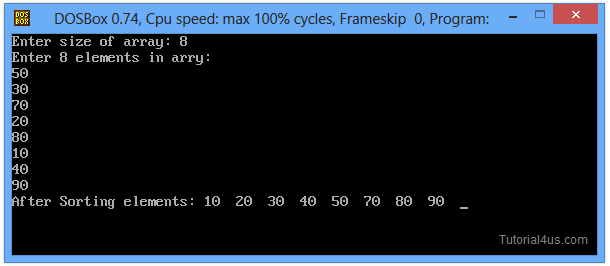# Insertion Sort in C++

## Insertion Sort Program in C++

Insertion Sort is a simplest data Sorting algorithm which sorts the array elements by shifting elements one by one and inserting each element into its proper position. This technique is also used for sort array elements. With the help of below animated image you can easily understand and you can also see real life example in second image.### Advantage of this sorting technique

It is a simple data Sorting algorithm. It is also better than Selection Sort and Bubble Sort algorithms.

### Dis-Advantage of this sorting technique

This sorting technique is slower than quick sort sorting algorithm.

## Insertion Sort Program in C

```
#include<stdio.h>
#include<conio.h>

void main()
{
int i, j, num, temp, arr;
clrscr();
cout<<"Enter size of array: ";
cin>>num;

cout<<"Enter "<<num <<elements in arry: \n";
for(i=0; i<num; i++)
{
cin>>arr[i];
}

for(i=1; i<num; i++)
{
temp=arr[i];
j=i-1;
while((temp<arr[j])&&(j>=0))
{
arr[j+1]=arr[j];
j=j-1;
}
arr[j+1]=temp;
}

cout<<"After Sorting elements: ";
for(i=0; i<num; i++)
{
cout<<"  "<< arr[i];
}
getch();
}
```

## Output

```
Enter size of array: 8
Enter 8 elements in arry
3
6
2
1
4
7
5
8
After Sorting elements: 1  2  3  4  5  6  7  8
```Note: Download above code and de-compress zip file, after that you can easily run this code.